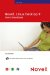# 118. About Calc Functions

 < Day Day Up >

Functions are built-in formulas that can do everything from add a range of values to provide an average for a range of cells to figure out the payment amount for a loan at a particular interest rate. Functions consist of two parts: the function name (such as =Average) and the range of cells that the function should act on. Calc provides many functions broken down into different categories (see Table 12.2). A statistical category includes functions such as average, maximum, and minimum. A financial category provides functions that allow you to calculate returns on investment and loan payment amounts among other finance-related calculations.

##### Table 12.2. Some of the Calc Function Categories

CATEGORY

EXAMPLE

PURPOSE

Date and Time

TODAY

Enters the current date

Financial

PMT

Calculates loan payments

Logical

IF

Returns a true or false answer to a conditional statement

Mathematical

COS

Calculates the cosine of an angle

Statistical

AVERAGE

Calculates the average for a range of cells

The easiest way to enter a function is through the Function AutoPilot. The AutoPilot allows you to select a particular function from the function list and then specify the cells that the function should act on. The AutoPilot also provides information about a selected function and makes it easy for you to get help as you work with a particular function.

 < Day Day Up >Novell Linux Desktop 9 Users Handbook
ISBN: 0672327295
EAN: 2147483647
Year: 2003
Pages: 244
Authors: Joe Habraken

flylib.com © 2008-2017.
If you may any questions please contact us: flylib@qtcs.net